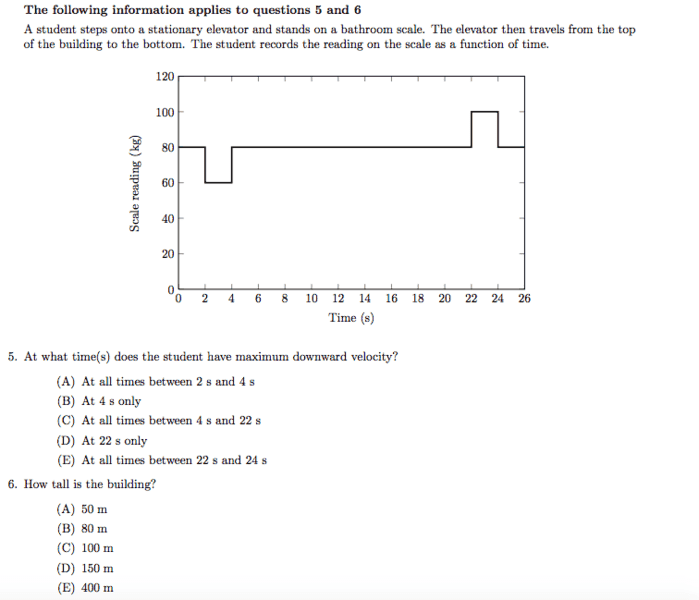# Area under the curve of a mass time graph

blueblast

## Homework Statement## Homework Equations

Force * time = mass * change in velocity[/B]

## The Attempt at a Solution

What I did was I converted the y-axis from kilograms to Newtons, since the "mass" reading is the force that the scale experiences. Then, the area under the curve will be the change in momentum, and if you divide that by the mass(80 kg, it is given that the elevator is stationary at t = 0), you can get the change in velocity. How would you use this to solve this problem? I know there are other ways to solve this, I'm just curious if my method works.

This is from the 2013 F=MA exam.

Thanks,

blueblast

andrewkirk
Homework Helper
Gold Member
You need to deduct the student's resting weight from the curve before measuring the area under it, as most of the force measured by the scale is being used to resist that weight.

Having done that, if you make a graph of the area under the curve against time (ie the integral of the graphed function), that will show momentum, and you'll be able to see where the period of maximum momentum is.

•blueblast
blueblast
You need to deduct the student's resting weight from the curve before measuring the area under it, as most of the force measured by the scale is being used to resist that weight.

Having done that, if you make a graph of the area under the curve against time (ie the integral of the graphed function), that will show momentum, and you'll be able to see where the period of maximum momentum is.

Could you possibly give me an example for one of the time periods?

andrewkirk
Homework Helper
Gold Member
Rather than go to all the trouble of calculating area under the graph, why not look at it and work out in what time period the lift is accelerating downwards, when it is accelerating upwards (ie slowing down), and when it is travelling at a constant rate. Think about yourself travelling in a lift. If it is going down, at what point in the ride do you feel lighter than usual, and at what point do you feel heavier than usual?

I love to jump in the air at these two times to feel the sensation of altered weight. is it just me or does anybody else like doing that?

blueblast
Rather than go to all the trouble of calculating area under the graph, why not look at it and work out in what time period the lift is accelerating downwards, when it is accelerating upwards (ie slowing down), and when it is travelling at a constant rate. Think about yourself travelling in a lift. If it is going down, at what point in the ride do you feel lighter than usual, and at what point do you feel heavier than usual?

I love to jump in the air at these two times to feel the sensation of altered weight. is it just me or does anybody else like doing that?

The only reason I actually want to get the values is because I solved it using a different way involving Newton's second law. That way seemed much more complicated than it needed to be, so I wanted to see if this method's values matched up with the more complicated way. I got the same results by subtracting the weight (800 N) from whatever value the y-axis displayed at the time(like you told me to do), then found the area under the curve. After that, I divided the area by the actual mass (80 kg), and got the same results as my other method :) Is this what you were suggesting initially?

Also, regarding your comment on jumping, I have always been afraid that the elevator cable will snap under my weight. A strange phobia of mine.

andrewkirk
•